# Python科学运算–简单汇总2019年3月30日20:40:58

4386字阅读14分37秒

## 矩阵运算

1. import numpy as np
2. x_test = np.array([[1,2,3],[3,4,6],[4,7,11]])
3. print(x_test)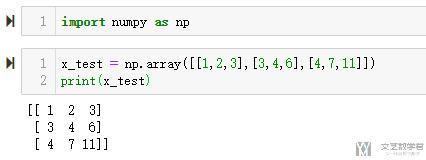### 矩阵转置

1. # 求矩阵转置
2. np.transpose(x_test)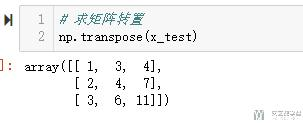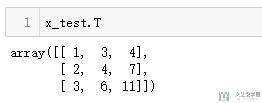### 矩阵求逆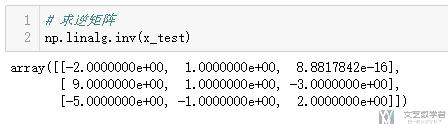### 矩阵求行列式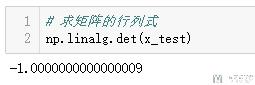### 矩阵求迹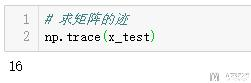### 生成全1矩阵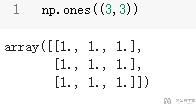### 生成对角矩阵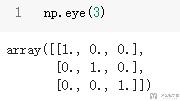### 特征值与特征向量

1. # 求矩阵的特征值与特征向量
2. w, v = np.linalg.eig(x_test)
3. print("Eigenvalues : \n{}".format(w)) # 特征值
4. print("Eigenvectors : \n{}".format(v)) # 特征向量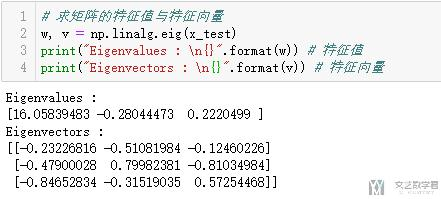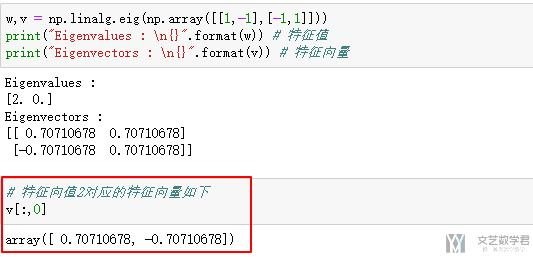### SVD分解

1. x_test = np.array([[1,2],[3,4],[5,6]])
2. # 进行svd分解
3. u,e,v = np.linalg.svd(x_test)
4. print("u:\n{}".format(u))
5. print("e:\n{}".format(e))
6. print("v:\n{}".format(v))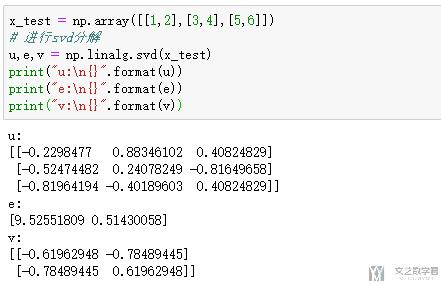1. # 将e矩阵补齐
2. e_n = np.zeros([3,2])
3. for i in range(2):
4.     e_n[i][i] = e[i]
5. print("u:\n{}".format(u))
6. print("e_n:\n{}".format(e_n))
7. print("v:\n{}".format(v))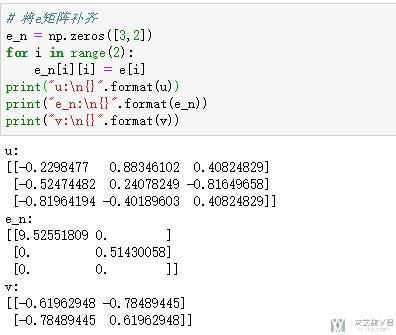1. # 恢复原矩阵做验证
2. np.dot(np.dot(u,e_n),
3.        v)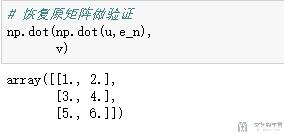### 矩阵乘法

1. x_test = np.array([[1,2,3],[4,5,6]])
2. np.dot(x_test, x_test.T) # 2*3 3*2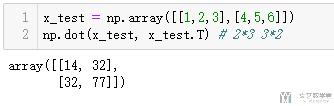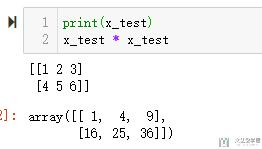## 统计相关

### 均值, 方差, 标准差

1. # 求均值
2. x_test = np.array([[1,2],[4,5],[7,8]])
3. x_test.mean(0)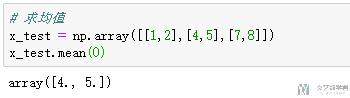1. # 求方差
2. x_test = np.array([[1,2],[4,5],[7,8]])
3. x_test.var(0)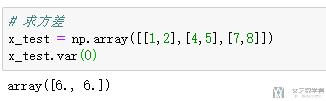1. # 求标准差
2. x_test = np.array([[1,2],[4,5],[7,8]])
3. x_test.std(0)
4. # np.sqrt(6)=2.44948974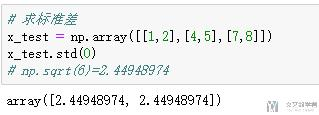### 协方差矩阵

1. # 计算方差矩阵
2. x_test = np.array([[1,1],[2,2],[3,3]])
3. np.cov(x_test.T) # 注意这里要进行转置, 这里维度是2, 有三个变量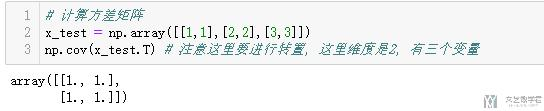### 相关系数矩阵

1. x_test = np.random.randint(2,10,(100,3)) # 随机生成一组数据
2. pd.DataFrame(x_test).corr() # 相关系数矩阵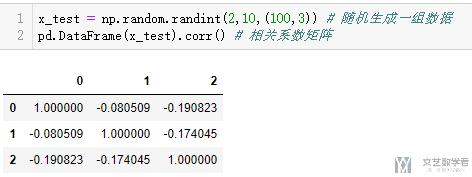### 协方差矩阵与相关系数矩阵可视化

1. import seaborn as sns
2. import matplotlib.pyplot as plt
3. %matplotlib inline
4. # 这里数据集是我之前的一个数据, 具体使用的时候进行修改即可
5. dfData = data_guess.iloc[:,:7].cov()
6. plt.subplots(figsize=(9, 9)) # 设置画面大小
7. sns.heatmap(dfData,square=True, cmap="Blues")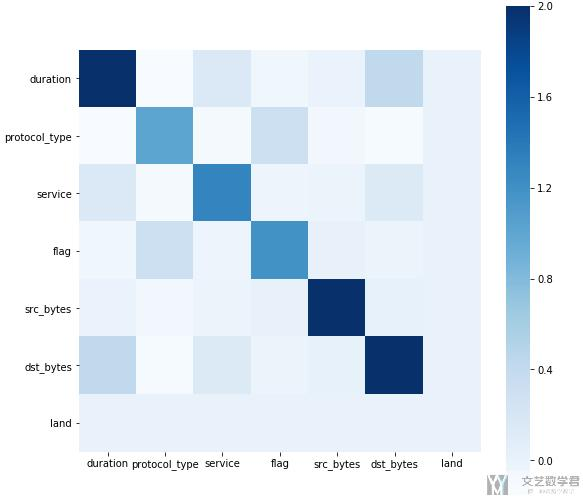## 数据预处理

1. from sklearn import preprocessing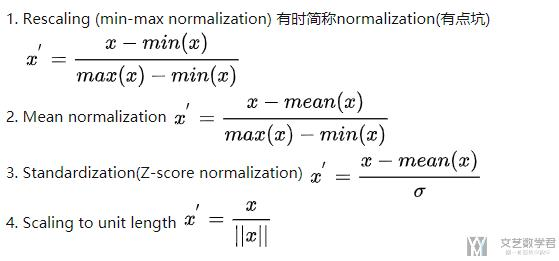### StandardScaler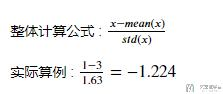1. x_tt = np.array([[1,2],[3,4],[5,6]])
2. preprocessing.scale(x_tt.astype(float))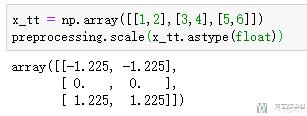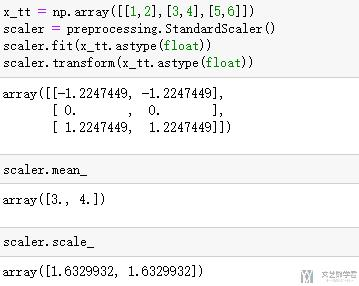### Normalize

1. x_tt = np.array([[1,2],[3,4],[5,6]])
2. preprocessing.normalize(x_tt.astype(float))
3. # 1/np.sqrt(1+4)=0.447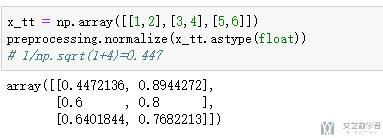### Label的生成

1. from sklearn import preprocessing
2. le = preprocessing.LabelEncoder()

1. le.fit(["paris", "paris", "tokyo", "amsterdam"])
2. # >> LabelEncoder()

1. list(le.classes_)
2. # >> ['amsterdam', 'paris', 'tokyo']

1. le.transform(["tokyo", "tokyo", "paris"])
2. # >> array([2, 2, 1]...)

1. list(le.inverse_transform([2, 2, 1]))
2. # >> ['tokyo', 'tokyo', 'paris']

### 离散变量变为标号(可以不用看, 使用上面的LabelEncoder代替)

1. test = pd.DataFrame(np.array([[1,'a'],[2,'b'],[3,'a']]),columns=['X1','X2'])
2. test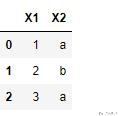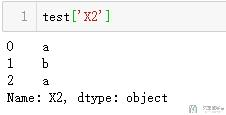1. test['X2']=test['X2'].astype('category')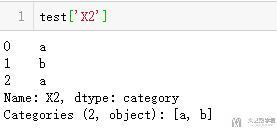1. test['X2']=test['X2'].cat.codes
2. test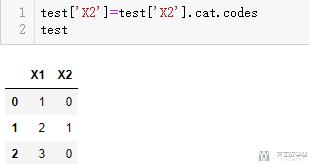1. test = pd.DataFrame(np.array([[1,'a'],[2,'b'],[3,'a']]),columns=['X1','X2'])
2. test = test.astype('category')
3. for i in test.columns:
4.     test[i] = test[i].cat.codes

### One-hot编码

1. # 转为one-hot编码
2. labels = ['a','b','c','d','d','a']
3. pd.get_dummies(labels)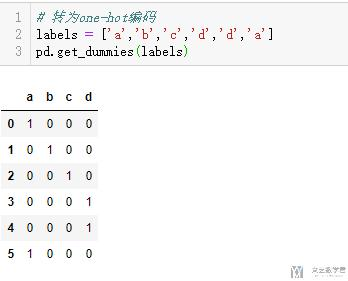• a: [0, 0]
• b: [1, 0]
• c: [0, 1]

1. labels = ['a','b','c','d','d','a']
2. pd.get_dummies(labels, drop_first=True)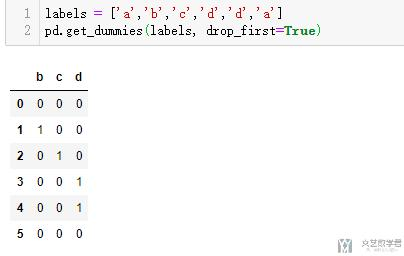### 关于One-hot的一点进阶说明

1. a = pd.DataFrame(np.array([[1.1,1.2,'a'],[2.9,2.0,'b'],[3,3,'a']]),columns=['X1','X2','X3'])
2. a['X1'] = a['X1'].astype(float)
3. a['X2'] = a['X2'].astype(float)
4. pd.get_dummies(a)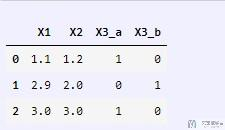## 抽样与乱序

1. data = pd.DaataFrame(np.array([[1,2],[3,4],[5,6]]),columns=['a','b'])
2. data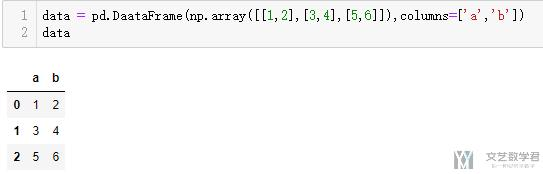### 抽样

1. data.sample(2)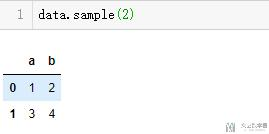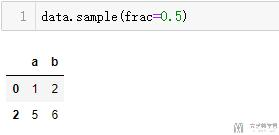### 乱序

1. data.sample(frac=1)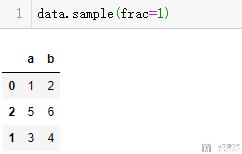1. test.reset_index(drop=True, inplace=True)

## 划分训练集和测试集

1. import numpy as np
2. from sklearn.model_selection import train_test_split
3. X_train, X_test, y_train, y_test = train_test_split(X, y, test_size=0.33, random_state=42)

• 微信公众号
• 关注微信公众号
•• QQ群
• 我们的QQ群号
•• 本文由 发表于 2019年3月30日20:40:58
• 转载请务必保留本文链接：https://mathpretty.com/10237.html